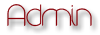EverEffects Backup ForumDesigners WorldShare |

# Scalar variablesAuthorMessage
Fred
StaffPosts : 33
Join date : 2010-05-27
Age : 26
Location : OklahomaSubject: Scalar variablesThu Jun 03, 2010 5:12 pmThis was dedicated Nik Silver as the first part of a one-day hands-on workshop at the School of Computer Studies, University of Leeds, in the UK. Who started Perl Language since 1990. Also i read his "Core Language" book, all 175 pages. So I learn so much in his book, you guys might think that reading is crap(that's what i think), but somehow the book become more interesting as i thought. So i will teach you about Operations and Assignment. I am using my own information and a little parts of the books information, also by using most of the books example but i will only clarify.So lets get started, now before we start learning about Operations and Assignment programming, I will teach you guys a little about "Scalar variables". Scalar variables are the most basic kind of variable in Perl. Scalar variables hold both strings and numbers, and are remarkable in that strings and numbers are completely interchangeable. For example, the statement:Now below me is the statementCode:\$priority = 9;sets the scalar variable \$priority to 9, but you can also assign a string to exactly the same variable:Code:\$priority = 'high';Perl also accepts numbers as strings, like this:Code:\$priority = '9';\$default = '0009';And can still cope with arithmetic and other operations quite happily.In general variable names consists of numbers, letters and underscores, but they should not start with a number and the variable \$_ is special, as we'll see later. Also, Perl is case sensitive, so \$a and \$A are different.Operations And AssignmentNow lets get started to Operations and Assignment.Perl uses all the usual C arithmetic operators:Code:\$a = 1 + 2; # Add 1 and 2 and store in \$a\$a = 3 - 4; # Subtract 4 from 3 and store in \$a\$a = 5 * 6; # Multiply 5 and 6\$a = 7 / 8; # Divide 7 by 8 to give 0.875\$a = 9 ** 10; # Nine to the power of 10\$a = 5 % 2; # Remainder of 5 divided by 2++\$a; # Increment \$a and then return it\$a++; # Return \$a and then increment it--\$a; # Decrement \$a and then return it\$a--; # Return \$a and then decrement itThis shows you math of Perl programming. And also for Perl strings has the following among others:Code:\$a = \$b . \$c; # Concatenate \$b and \$c\$a = \$b x \$c; # \$b repeated \$c timesHere is a source for assign values Perl includes:Code:\$a = \$b; # Assign \$b to \$a\$a += \$b; # Add \$b to \$a\$a -= \$b; # Subtract \$b from \$a\$a .= \$b; # Append \$b onto \$aThe following code prints apples and pears using concatenation:Interpolation\$a = 'apples';\$b = 'pears';print \$a.' and '.\$b;It would be nicer to include only one string in the final print statement, but the lineprint '\$a and \$b';prints literally \$a and \$b which isn't very helpful. Instead we can use the double quotes in place of the single quotes:print "\$a and \$b";The double quotes force interpolation of any codes, including interpreting variables. This is a much nicer than our original statement. Other codes that are interpolated include special characters such as newline and tab. The code \n is a newline and \t is a tab. Note that when Perl assigns a value with \$a = \$b it makes a copy of \$b and then assigns that to \$a. Therefore the next time you change \$b it will not alter \$a.Thanks for reading and i hope you learn somethingFeel free to ask me questions and also for more Perl tutorials# Scalar variablesPage 1 of 1

Permissions in this forum:You cannot reply to topics in this forum